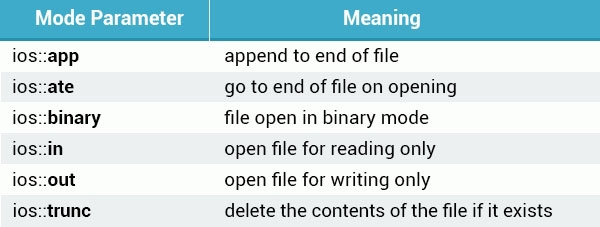# C++ 入门教程之八 -- Templates - Exceptions - Files

### function template

``````int sum(int a, int b) {
return a+b;
}

int main () {
int x=7, y=15;
cout << sum(x, y) << endl;
}``````

`sum()` 可以正确的执行指令，但它的限制是必须传入 int 类型的数据。

``````double sum(double a, double b) {
return a+b;
}``````

``````template <class T>
``````

``````template <typename T>
``````

`T` 表示我们的 generic 通用数据类型名称，在后续中可以使用此名称代表数据类型，可以是任意自定义字符

``````#include <iostream>
using namespace std;

template <class T>
T sum(T a, T b)
{
return a + b;
}

int main()
{
int x=7, y=15;
cout << sum(x, y) << endl;

double a=7.15, b=15.54;
cout << sum(a, b) << endl;

return 0;
}``````

function template 可以节省编程中很多时间，因为只需要一次定义就可以兼容不同的数据类型。

``````template <class T, class U>
T smaller(T a, U b) {
return (a < b ? a : b);
}

int main () {
int x=72;
double y=15.34;
cout << smaller(x, y) << endl;
}``````

### class template

``````template <class T>
class MyClass {

};``````

``````template <class T,class U>
``````

``````template <class T>
class Pair {
public:
Pair (T a, T b):
first(a), second(b) {
}

private:
T first, second;
};``````

``````template <class T>
class Pair {
public:
Pair (T a, T b):
first(a), second(b) {
}
T bigger();

private:
T first, second;
};

template <class T>
T Pair<T>::bigger() {
return (first>second ? first : second);
}``````

bigger function 返回 class 两个属性变量中较大的值。

``````void main() {
Pair<int> obj(11, 22);
cout << obj.bigger() << endl;
}``````

``````void main() {
Pair<double> obj(11.23, 22.56);
cout << obj.bigger() << endl;
}``````

### template 特例

``````template <class T>
class MyClass {
public:
MyClass(T x) {
cout << x << " - not a char" << endl;
}
};``````

``````template <>
class MyClass<char> {
public:
MyClass(char x) {
cout << x << " - is a char" << endl;
}
};``````

``````int main() {
MyClass<int> obj1(22);
MyClass<double> obj2(11.34);
MyClass<char> obj3('a');

return 0;
}

//output:
//22 - not a char
//11.34 - not a char
//a - is a char``````

### exception 例外

c++ 的 exception handling 例外管理器是通过三个关键词：`try``catch``throw` 来建立的。`throw` 是用来当问题出现时调出某一个 exception 响应动作的。例如：

``````int motherAge = 40;
int sonAge = 50;
if (sonAge > motherAge) {
throw 99;
}``````

throw 中的数据类型可以是任意的，如 123 为 int 型，a 为 char 型，11.24 为 double 型，一下示例写法都是正确的：

``````int motherAge = 40;
int sonAge = 50;
if (sonAge > motherAge) {
throw ‘a’;
}

if (sonAge = motherAge) {
throw 12.45;
}``````

### catching exception

``````try {
int motherAge = 40;
int sonAge = 50;
if (sonAge > motherAge) {
throw 99;
}
} catch (int x) {
cout << "wrong age value - Error" << x << endl;
} catch (char x) {
cout << "example for other catch" << x << endl;
}

//output: wrong age value - Error99``````

``````try {
int num1;
cout << "enter the first number:";
cin >> num1;

int num2;
cout << "enter the second number:";
cin >> num2;

if (num2 == 0) {
throw 0;
}
cout << "result: " << num1 / num2 << endl;
} catch (int x) {
cout << "division by zero!" << endl;
}``````

`cin` 是 istream class 类型的 object 用来输入数据流，数据输入后会存在后面的变量中。当输入的第二个数字非零时不会触发 exception 响应，当为零时 throw 类型为 int，然后匹配到 exception 中类型也为 int 的块，然后执行其中指令。

catch 块也可以管理在 try 块中任何类型的 throw exception，不同于catch 后定义某一种数据类型，使用省略号`(...)` 来表示响应任何类型的 throw，例如：

``````try {
//...
} catch (...) {
cout << "division by zero!" << endl;
}``````

### Files 文件处理

c++ 另一个常用的功能就是对文件的读写操作，需要用到 c++ 标准库：`'

• ofstream：输出文件流，用来创建或写入文件
• ifstream：输入文件流，用来读取文件信息
• fstream：通用文件流，包含 ofsteam 和 ifstream 的内容，支持创建，读入，写入文件。

``````#include <iostream>
#include <fstream>``````

### 打开文件及写入

ofstream 和 fstream 的 object 都可以用来打开文件然后进行写操作，我们打开一个 test.txt 文件然后写入内容最后关闭文件：

``````#include <iostream>
#include <fstream>
using namespace std;

int main() {
ofstream MyFile;
MyFile.open("test.txt");
MyFile << "some text.\n";
MyFile.close();

return 0;
}``````

open function 打开的文件可以是一个路径，如果只有文件名则默认在程序根目录。

``````int main(int argc, const char * argv[]) {

ofstream MyFile("test.txt");
MyFile << "some text.\n";
MyFile.close();

return 0;
}``````

``````int main(int argc, const char * argv[]) {

ofstream MyFile("test.txt");
if (MyFile.is_open()) {
MyFile << "some text.\n";
} else {
cout << "somethin went wrong" << endl;
}
MyFile.close();

return 0;
}``````

is_open function 检查文件是否被正常打开，返回值为：true 或 false。

open function 的第二参数可以用来定义文件打开模式，一下是支持的模式列表：``````ofstream outfile;
outfile.open("file.dat", ios::out | ios::trunc );``````

### 读取文件

``````string line;